|

# 优梵艺术设计师沙龙 兼具调性与温度 推动国内外设计行业交流

3月9日，时值优梵艺术杭州艺术生活馆开业，优梵艺术在第六空间西溪艺术生活广场举办主题为“聚设计·新品牌·梵计划”的设计师分享沙龙。优梵艺术董事长林上康（Jacky）和意大利知名设计师、优梵艺术艺术总监、UVANART curated by Luca Boffi子品牌总设计师Luca Boffi，以及国内外设计大咖Gabreiele Cappelltti、Paolo Gerosa、Manuela Bucci、杭州一路文化创始人王晴、第六空间城西店总经理王怡等齐聚活动现场，共同围绕国内外设计文化和理念展开深度的思维碰撞与艺术交流。Luca在致辞中表示：“ ‘让平民也能拥有伟大的设计’是我的梦想，我希望做具有艺术观赏、实用设计，与大众消费水平相结合的产品。为此，我也一直在努力，并且拥有了我的个人品牌Luca boffi，很多非常著名的设计师愿意与我合作。我对中国一直充满着敬意，当我遇到Jacky，他告诉我优梵艺术正在做的事情——让家具设计成为一种艺术，让更多人拥有其向往的美好生活。这与我一直在意大利做的是一致的，正因如此，我选择和优梵艺术一起，在中国做买得起的艺术家具。UVANART curated by Luca Boffi是我们合作的第一个子品牌，我们将会把它做到极致，让它走向世界，把美好传递给更多人。”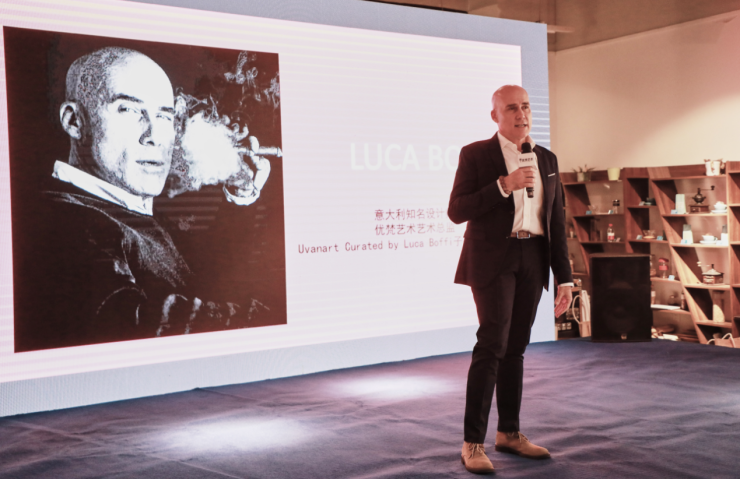UVANART curated by Luca Boffi 品牌总设计师Luca Boffi讲解品牌创立的初衷Paolo有着丰富的设计经历，在设计学府PoliMi毕业后，加⼊知名的LPWK设计工作室，开始了与意大利家居品牌Alessi的设计合作，并设计了多款畅销产品。2015年为米兰世博会意大利国家馆设计“冰淇淋与巧克力”主题展区。在介绍部分设计作品之后，POLO现场也提到：“设计一个产品最有趣和最有价值的一个方面是观察产品与人之间的相关性。” 据悉，Paolo在2017年就为优梵艺术设计过Lavita城市生活系列产品。该系列新颖的设计灵感，延续了2017年最新为Alessi设计的“shot”元素，针对青年人对于个性和美的需求，打造专属的居住卧室，也赢得了中国年轻消费者的喜爱。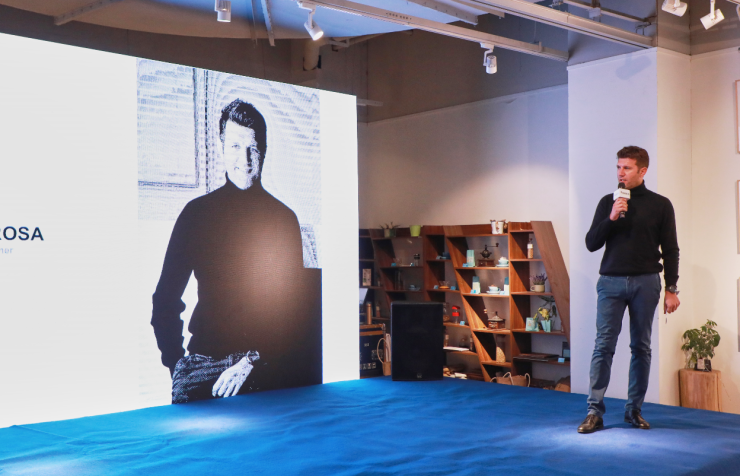UVANART x MFDS LaVita 卧室系列设计师Paolo Gerosa与现场设计师分享其设计思想UVANART x MFDS LaVita 卧室系列

Gabriele是意大利建筑设计师、设计师，其主要从事室内设计、产品设计以及建筑设计。从业至今，他在世界各地参与了多个国际项目的设计，同时与多个大型家具企业、拥有超高技艺的工匠，保持着非常友好且密切的合作与沟通。作为一个年轻设计师，他的作品得了多个奖项，包括：2013 米兰装饰设计大奖、2016芝加哥优秀设计大奖、以及2018、2019连续两年获得德国设计大奖等。

Gabriele现场分享他所积累的丰富设计经验，最大的特色在于注重材料。“材料直接促成了我的成功，材料就是设计的工具，是所有设计的第一步，直接决定设计成败的关键” Gabriele非常认真地讲到。也就是因为注重这些设计材料的准确性，包括设计细节上完美把控，他的作品才闻名于世。Manuela Bucci自2000年在米兰理工大学工业设计专业后便加入Cini Boeri，在创作中，她擅长将人类习惯与技术相结合，并以非常个性化的肖像概念挖掘主题的特点。Manuela以最佳的设计概念赢得红点设计大奖评审的亲睐，成功斩获2017红点设计概念大奖。

Manuela设计领域非常广泛，包括手机、家电、饰品等，这些跨界的设计经验，为她在家具的个性化、功能性、互动性设计概念积累了良好的基础。同时，Manuela也是一个非常注重材料的设计师：“因为这不仅关乎到产品的质量，还有一个品牌的调性，甚至是市场的推广，所以我在设计一件产品的时候，是全程参与到每一个环节，从设计，选材、打样、成品等。 ”在分享的最后，Luca为大家宣导了设计与艺术之间互通性的观念，同时他认为Manuela在某种意义上可称为艺术家，而其创造的大部分作品，已是艺术品。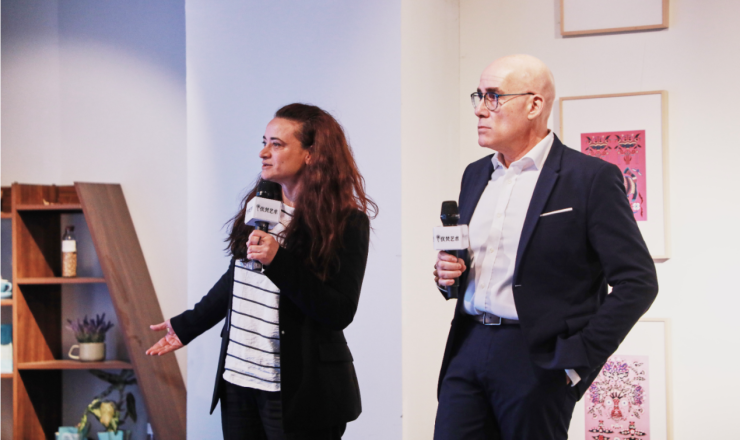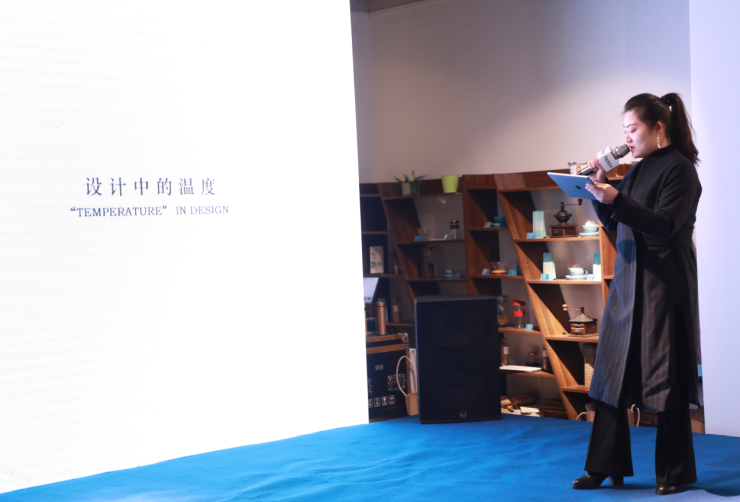“设计师合伙人招募计划”发布 助力设计行业发展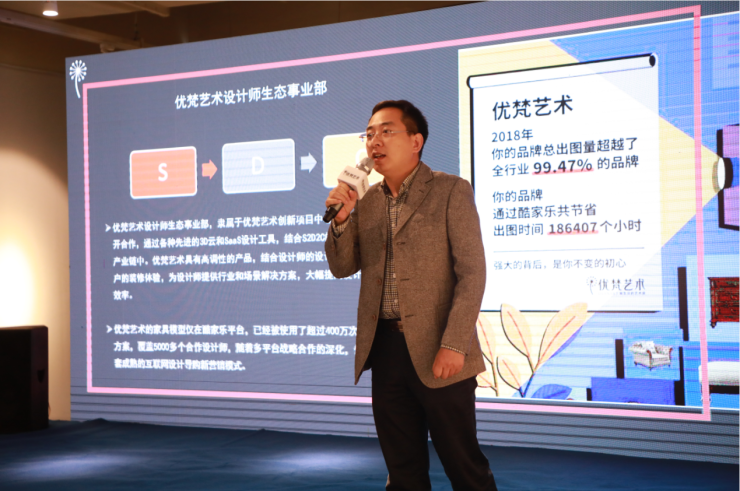“设计来源于生活，亦高于生活，设计行业的发展影响着美好生活的建设”，第六空间城西店总经理王怡作为平台方，现场就设计方面也发表了独特见解，这是一场关于设计生活的实践探索，也是一段追寻家居空间多样性与艺术性的创新旅程，作为一个时尚高端家居平台，第六空间愿意为这些优秀的设计、优秀的品牌提供更多的展示机会。

`声明：本文由入驻焦点开放平台的作者撰写，除焦点官方账号外，观点仅代表作者本人，不代表焦点立场错误信息举报电话： 400-099-0099，邮箱：jubao@vip.sohu.com，或点此进行意见反馈，或点此进行举报投诉。`A B C D E F G H J K L M N P Q R S T W X Y Z
A - B - C - D - E
• A
• 鞍山
• 安庆
• 安阳
• 安顺
• 安康
• 澳门
• B
• 北京
• 保定
• 包头
• 巴彦淖尔
• 本溪
• 蚌埠
• 亳州
• 滨州
• 北海
• 百色
• 巴中
• 毕节
• 保山
• 宝鸡
• 白银
• 巴州
• C
• 承德
• 沧州
• 长治
• 赤峰
• 朝阳
• 长春
• 常州
• 滁州
• 池州
• 长沙
• 常德
• 郴州
• 潮州
• 崇左
• 重庆
• 成都
• 楚雄
• 昌都
• 慈溪
• 常熟
• D
• 大同
• 大连
• 丹东
• 大庆
• 东营
• 德州
• 东莞
• 德阳
• 达州
• 大理
• 德宏
• 定西
• 儋州
• 东平
• E
• 鄂尔多斯
• 鄂州
• 恩施
F - G - H - I - J
• F
• 抚顺
• 阜新
• 阜阳
• 福州
• 抚州
• 佛山
• 防城港
• G
• 赣州
• 广州
• 桂林
• 贵港
• 广元
• 广安
• 贵阳
• 固原
• H
• 邯郸
• 衡水
• 呼和浩特
• 呼伦贝尔
• 葫芦岛
• 哈尔滨
• 黑河
• 淮安
• 杭州
• 湖州
• 合肥
• 淮南
• 淮北
• 黄山
• 菏泽
• 鹤壁
• 黄石
• 黄冈
• 衡阳
• 怀化
• 惠州
• 河源
• 贺州
• 河池
• 海口
• 红河
• 汉中
• 海东
• I
• J
• 晋中
• 锦州
• 吉林
• 鸡西
• 佳木斯
• 嘉兴
• 金华
• 景德镇
• 九江
• 吉安
• 济南
• 济宁
• 焦作
• 荆门
• 荆州
• 江门
• 揭阳
• 金昌
• 酒泉
• 嘉峪关
K - L - M - N - P
• K
• 开封
• 昆明
• 昆山
• L
• 廊坊
• 临汾
• 辽阳
• 连云港
• 丽水
• 六安
• 龙岩
• 莱芜
• 临沂
• 聊城
• 洛阳
• 漯河
• 娄底
• 柳州
• 来宾
• 泸州
• 乐山
• 六盘水
• 丽江
• 临沧
• 拉萨
• 林芝
• 兰州
• 陇南
• M
• 牡丹江
• 马鞍山
• 茂名
• 梅州
• 绵阳
• 眉山
• N
• 南京
• 南通
• 宁波
• 南平
• 宁德
• 南昌
• 南阳
• 南宁
• 内江
• 南充
• P
• 盘锦
• 莆田
• 平顶山
• 濮阳
• 攀枝花
• 普洱
• 平凉
Q - R - S - T - W
• Q
• 秦皇岛
• 齐齐哈尔
• 衢州
• 泉州
• 青岛
• 清远
• 钦州
• 黔南
• 曲靖
• 庆阳
• R
• 日照
• 日喀则
• S
• 石家庄
• 沈阳
• 双鸭山
• 绥化
• 上海
• 苏州
• 宿迁
• 绍兴
• 宿州
• 三明
• 上饶
• 三门峡
• 商丘
• 十堰
• 随州
• 邵阳
• 韶关
• 深圳
• 汕头
• 汕尾
• 三亚
• 三沙
• 遂宁
• 山南
• 商洛
• 石嘴山
• T
• 天津
• 唐山
• 太原
• 通辽
• 铁岭
• 泰州
• 台州
• 铜陵
• 泰安
• 铜仁
• 铜川
• 天水
• 天门
• W
• 乌海
• 乌兰察布
• 无锡
• 温州
• 芜湖
• 潍坊
• 威海
• 武汉
• 梧州
• 渭南
• 武威
• 吴忠
• 乌鲁木齐
X - Y - Z
• X
• 邢台
• 徐州
• 宣城
• 厦门
• 新乡
• 许昌
• 信阳
• 襄阳
• 孝感
• 咸宁
• 湘潭
• 湘西
• 西双版纳
• 西安
• 咸阳
• 西宁
• 仙桃
• 西昌
• Y
• 运城
• 营口
• 盐城
• 扬州
• 鹰潭
• 宜春
• 烟台
• 宜昌
• 岳阳
• 益阳
• 永州
• 阳江
• 云浮
• 玉林
• 宜宾
• 雅安
• 玉溪
• 延安
• 榆林
• 银川
• Z
• 张家口
• 镇江
• 舟山
• 漳州
• 淄博
• 枣庄
• 郑州
• 周口
• 驻马店
• 株洲
• 张家界
• 珠海
• 湛江
• 肇庆
• 中山
• 自贡
• 资阳
• 遵义
• 昭通
• 张掖
• 中卫

1室1厅1厨1卫1阳台

1
2
3
4
5

0
1
2

1

1

0
1
2
3报名成功，资料已提交审核A B C D E F G H J K L M N P Q R S T W X Y Z
A - B - C - D - E
• A
• 鞍山
• 安庆
• 安阳
• 安顺
• 安康
• 澳门
• B
• 北京
• 保定
• 包头
• 巴彦淖尔
• 本溪
• 蚌埠
• 亳州
• 滨州
• 北海
• 百色
• 巴中
• 毕节
• 保山
• 宝鸡
• 白银
• 巴州
• C
• 承德
• 沧州
• 长治
• 赤峰
• 朝阳
• 长春
• 常州
• 滁州
• 池州
• 长沙
• 常德
• 郴州
• 潮州
• 崇左
• 重庆
• 成都
• 楚雄
• 昌都
• 慈溪
• 常熟
• D
• 大同
• 大连
• 丹东
• 大庆
• 东营
• 德州
• 东莞
• 德阳
• 达州
• 大理
• 德宏
• 定西
• 儋州
• 东平
• E
• 鄂尔多斯
• 鄂州
• 恩施
F - G - H - I - J
• F
• 抚顺
• 阜新
• 阜阳
• 福州
• 抚州
• 佛山
• 防城港
• G
• 赣州
• 广州
• 桂林
• 贵港
• 广元
• 广安
• 贵阳
• 固原
• H
• 邯郸
• 衡水
• 呼和浩特
• 呼伦贝尔
• 葫芦岛
• 哈尔滨
• 黑河
• 淮安
• 杭州
• 湖州
• 合肥
• 淮南
• 淮北
• 黄山
• 菏泽
• 鹤壁
• 黄石
• 黄冈
• 衡阳
• 怀化
• 惠州
• 河源
• 贺州
• 河池
• 海口
• 红河
• 汉中
• 海东
• I
• J
• 晋中
• 锦州
• 吉林
• 鸡西
• 佳木斯
• 嘉兴
• 金华
• 景德镇
• 九江
• 吉安
• 济南
• 济宁
• 焦作
• 荆门
• 荆州
• 江门
• 揭阳
• 金昌
• 酒泉
• 嘉峪关
K - L - M - N - P
• K
• 开封
• 昆明
• 昆山
• L
• 廊坊
• 临汾
• 辽阳
• 连云港
• 丽水
• 六安
• 龙岩
• 莱芜
• 临沂
• 聊城
• 洛阳
• 漯河
• 娄底
• 柳州
• 来宾
• 泸州
• 乐山
• 六盘水
• 丽江
• 临沧
• 拉萨
• 林芝
• 兰州
• 陇南
• M
• 牡丹江
• 马鞍山
• 茂名
• 梅州
• 绵阳
• 眉山
• N
• 南京
• 南通
• 宁波
• 南平
• 宁德
• 南昌
• 南阳
• 南宁
• 内江
• 南充
• P
• 盘锦
• 莆田
• 平顶山
• 濮阳
• 攀枝花
• 普洱
• 平凉
Q - R - S - T - W
• Q
• 秦皇岛
• 齐齐哈尔
• 衢州
• 泉州
• 青岛
• 清远
• 钦州
• 黔南
• 曲靖
• 庆阳
• R
• 日照
• 日喀则
• S
• 石家庄
• 沈阳
• 双鸭山
• 绥化
• 上海
• 苏州
• 宿迁
• 绍兴
• 宿州
• 三明
• 上饶
• 三门峡
• 商丘
• 十堰
• 随州
• 邵阳
• 韶关
• 深圳
• 汕头
• 汕尾
• 三亚
• 三沙
• 遂宁
• 山南
• 商洛
• 石嘴山
• T
• 天津
• 唐山
• 太原
• 通辽
• 铁岭
• 泰州
• 台州
• 铜陵
• 泰安
• 铜仁
• 铜川
• 天水
• 天门
• W
• 乌海
• 乌兰察布
• 无锡
• 温州
• 芜湖
• 潍坊
• 威海
• 武汉
• 梧州
• 渭南
• 武威
• 吴忠
• 乌鲁木齐
X - Y - Z
• X
• 邢台
• 徐州
• 宣城
• 厦门
• 新乡
• 许昌
• 信阳
• 襄阳
• 孝感
• 咸宁
• 湘潭
• 湘西
• 西双版纳
• 西安
• 咸阳
• 西宁
• 仙桃
• 西昌
• Y
• 运城
• 营口
• 盐城
• 扬州
• 鹰潭
• 宜春
• 烟台
• 宜昌
• 岳阳
• 益阳
• 永州
• 阳江
• 云浮
• 玉林
• 宜宾
• 雅安
• 玉溪
• 延安
• 榆林
• 银川
• Z
• 张家口
• 镇江
• 舟山
• 漳州
• 淄博
• 枣庄
• 郑州
• 周口
• 驻马店
• 株洲
• 张家界
• 珠海
• 湛江
• 肇庆
• 中山
• 自贡
• 资阳
• 遵义
• 昭通
• 张掖
• 中卫• 手机• 分享
• 设计
免费设计
• 计算器
装修计算器
• 入驻
合作入驻
• 联系
联系我们
• 置顶
返回顶部